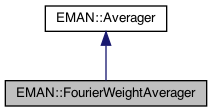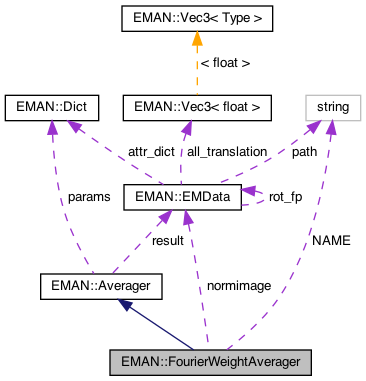EMAN2
EMAN::FourierWeightAverager Class Reference

FourierWeightAverager makes an average of a set of images in Fourier space using a per-image radial weight. More...

`#include <averager.h>`

Inheritance diagram for EMAN::FourierWeightAverager:[legend]
Collaboration diagram for EMAN::FourierWeightAverager:[legend]

## Public Member Functions

FourierWeightAverager ()

To add an image to the Averager. More...

EMDatafinish ()
Finish up the averaging and return the result. More...

string get_name () const
Get the Averager's name. More...

string get_desc () const

TypeDict get_param_types () const
Get Averager parameter information in a dictionary. More...Public Member Functions inherited from EMAN::Averager
Averager ()

virtual ~Averager ()

virtual void add_image_list (const vector< EMData * > &images)
To add multiple images to the Averager. More...

virtual void set_params (const Dict &new_params)
Set the Averager parameters using a key/value dictionary. More...

virtual void mult (const float &s)
Multiply the result image by some floating point constant This is useful when weighting the input images prior to calling add_image - a situation where it is likely you want to divide by the sum of the weights. More...

## Static Public Member Functions

static AveragerNEW ()

## Static Public Attributes

static const string NAME = "weightedfourier"

## Private Attributes

EMDatanormimage

int freenorm

int nimgProtected Attributes inherited from EMAN::Averager
Dict params

EMDataresult

## Detailed Description

FourierWeightAverager makes an average of a set of images in Fourier space using a per-image radial weight.

The provided XYData object for each inserted image should range from x=0 - 0.5*sqrt(2), and contains the radial weights from 0 - Nyquist at the point. If the x range is insufficient, values will be clamped at the ends of the available x-range. 2-D Images only, but will work with rectangular images.

Parameters
 normimage After finish() will contain the sum of the weights in each Fourier location. Size must be ((nx+1)/2,y)

Definition at line 301 of file averager.h.

## ◆ FourierWeightAverager()

 FourierWeightAverager::FourierWeightAverager ( )

Definition at line 462 of file averager.cpp.

Referenced by NEW().

## Member Function Documentation

 void FourierWeightAverager::add_image ( EMData * image )
virtual

To add an image to the Averager.

This image will be averaged in this function.

Parameters
 image The image to be averaged.

Reimplemented from EMAN::Averager.

Definition at line 468 of file averager.cpp.

469{
470 if (!image) {
471 return;
472 }
473
474
475
476 EMData *img=image->do_fft();
477 if (nimg >= 1 && !EMUtil::is_same_size(img, result)) {
478 LOGERR("%sAverager can only process same-size Image",
479 get_name().c_str());
480 return;
481 }
482
483 nimg++;
484
485 int nx = img->get_xsize();
486 int ny = img->get_ysize();
487 int nz = 1;
488// size_t image_size = (size_t)nx * ny * nz;
489
490 XYData *weight=(XYData *)image->get_attr("avg_weight");
491
492 if (nimg == 1) {
493 result = new EMData(nx,ny,nz);
494 result->set_complex(true);
495 result->to_zero();
496
497 normimage = params.set_default("normimage", (EMData*)0);
498 if (normimage==0) { normimage=new EMData(nx/2,ny,nz); freenorm=1; }
499 normimage->to_zero();
500 }
501
502 // We're using routines that handle complex image wraparound for us, so we iterate over the half-plane
503 for (int y=-ny/2; y<ny/2; y++) {
504 for (int x=0; x<nx/2; x++) {
505 std::complex<float> v=img->get_complex_at(x,y);
506 float r=Util::hypot2(y/(float)ny,x/(float)nx);
507 float wt=weight->get_yatx(r);
508 result->set_complex_at(x,y,result->get_complex_at(x,y)+v*wt);
509 normimage->set_value_at(x,y+ny/2,normimage->get_value_at(x,y+ny/2)+wt);
510 }
511 }
512
513 delete img;
514}
EMData * result
Definition: averager.h:158
type set_default(const string &key, type val)
Default setting behavior This can be achieved using a template - d.woolford Jan 2008 (before there wa...
Definition: emobject.h:569
EMData stores an image's data and defines core image processing routines.
Definition: emdata.h:82
static bool is_same_size(const EMData *image1, const EMData *image2)
Check whether two EMData images are of the same size.
Definition: emutil.cpp:1224
string get_name() const
Get the Averager's name.
Definition: averager.h:309
static float hypot2(float x, float y)
Euclidean distance function in 2D: f(x,y) = sqrt(x*x + y*y);.
Definition: util.h:774
XYData defines a 1D (x,y) data set.
Definition: xydata.h:47
float get_yatx(float x, bool outzero=true)
Definition: xydata.cpp:172
#define LOGERR
Definition: log.h:51
#define y(i, j)
Definition: projector.cpp:1516
#define x(i)
Definition: projector.cpp:1517

## ◆ finish()

 EMData * FourierWeightAverager::finish ( )
virtual

Finish up the averaging and return the result.

Returns
The averaged image.

Implements EMAN::Averager.

Definition at line 516 of file averager.cpp.

517{
518 EMData *ret = (EMData *)0;
519
520 if (result && nimg >= 1) {
521 // We're using routines that handle complex image wraparound for us, so we iterate over the half-plane
522 int nx = result->get_xsize();
523 int ny = result->get_ysize();
524
525 for (int y=-ny/2; y<ny/2; y++) {
526 for (int x=0; x<nx/2; x++) {
527 float norm=normimage->get_value_at(x,y+ny/2);
528 if (norm<=0) result->set_complex_at(x,y,0.0f);
529 else result->set_complex_at(x,y,result->get_complex_at(x,y)/norm);
530 }
531 }
532
533 result->update();
534// result->mult(1.0f/(float)result->get_attr("sigma"));
535// result->write_image("tmp.hdf",0);
536// printf("%g %g %g\n",(float)result->get_attr("sigma"),(float)result->get_attr("minimum"),(float)result->get_attr("maximum"));
537 ret=result->do_ift();
538 delete result;
539 result=(EMData*) 0;
540 }
541 ret->set_attr("ptcl_repr",nimg);
542
543 if (freenorm) { delete normimage; normimage=(EMData*)0; }
544 nimg=0;
545
546 return ret;
547}

References freenorm, nimg, normimage, EMAN::Averager::result, x, and y.

## ◆ get_desc()

 string EMAN::FourierWeightAverager::get_desc ( ) const
inlinevirtual

Implements EMAN::Averager.

Definition at line 314 of file averager.h.

315 {
316 return "Weighted mean of images in Fourier space. Each image must have weighting curve in its header, an XYData object called 'avg_weight'.";
317 }

## ◆ get_name()

 string EMAN::FourierWeightAverager::get_name ( ) const
inlinevirtual

Get the Averager's name.

Each Averager is identified by a unique name.

Returns
The Averager's name.

Implements EMAN::Averager.

Definition at line 309 of file averager.h.

310 {
311 return NAME;
312 }
static const string NAME
Definition: averager.h:332

References NAME.

## ◆ get_param_types()

 TypeDict EMAN::FourierWeightAverager::get_param_types ( ) const
inlinevirtual

Get Averager parameter information in a dictionary.

Each parameter has one record in the dictionary. Each record contains its name, data-type, and description.

Returns
A dictionary containing the parameter info.

Reimplemented from EMAN::Averager.

Definition at line 324 of file averager.h.

325 {
326 TypeDict d;
327// d.put("weight", EMObject::XYDATA, "Radial weight. X: 0 - 0.5*sqrt(2). Y contains weights.");
328 d.put("normimage", EMObject::EMDATA, "After finish() will contain the sum of the weights in each Fourier location. Size must be ((nx+1)/2,y)");
329 return d;
330 }

References EMAN::EMObject::EMDATA, and EMAN::TypeDict::put().

## ◆ NEW()

 static Averager * EMAN::FourierWeightAverager::NEW ( )
inlinestatic

Definition at line 319 of file averager.h.

320 {
321 return new FourierWeightAverager();
322 }

References FourierWeightAverager().

## ◆ freenorm

 int EMAN::FourierWeightAverager::freenorm
private

Definition at line 336 of file averager.h.

## ◆ NAME

 const string FourierWeightAverager::NAME = "weightedfourier"
static

Definition at line 332 of file averager.h.

Referenced by get_name().

## ◆ nimg

 int EMAN::FourierWeightAverager::nimg
private

Definition at line 337 of file averager.h.